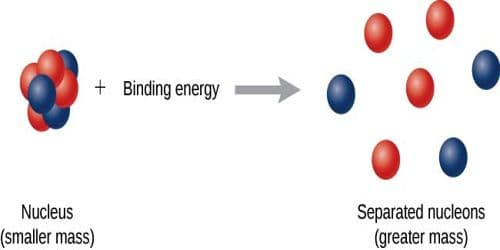Physics

# Binding EnergyBinding Energy

We know, every nucleus, except hydrogen is composed of one or more proton and neutron. These protons and neutrons are called nucleons or nuclear elements. Combining nucleons or nuclear elements to form a permanent nucleus some amount of energy is emitted. This energy is equal to the equivalent energy of mass-defect. Again, to break a nucleus into its separate nucleons, some energy from outside is needed to supply. This energy is called binding energy or nuclear binding energy. From the above discussion, we can define the binding energy in the following way.

(a) Amount of energy released in order to form a permanent nucleus by combining the necessary number of nucleons is called binding energy. It is expressed as B.E.

(b) The amount of energy supplied to the nucleus to separate the constituent nucleons which become free from their mutual influence is called the binding energy.

It is to be mentioned, that nuclear binding energy is originated train nuclear force acting to keep the nucleons together and it is responsible for the stability of the nucleus. If the binding energy is more, the nucleus is more stable.

Suppose mass defect of the nucleus = Δm, and velocity of light is c. So from Einstein’s theory of relativity, we get,

Binding energy, B.E. = Δmc2 = mass defect x (velocity of light)2

or, B. E. = [ZMP + (A — Z)Mn — M] c2

This is the equation for the nuclear binding energy.

[N. B. For the binding energy another equation can be used, which is B. E. = Δm.c2 = [(M – A) c2]

Example: a Mass defect of the nucleus of the deuteron,

Δm = 0.002388 a.m.u.

But, 1 a.m.u. = 931 MeV

So, Binding energy of the deuteron is. B.E. = 0.002388 x 931 = 2.23 MeV